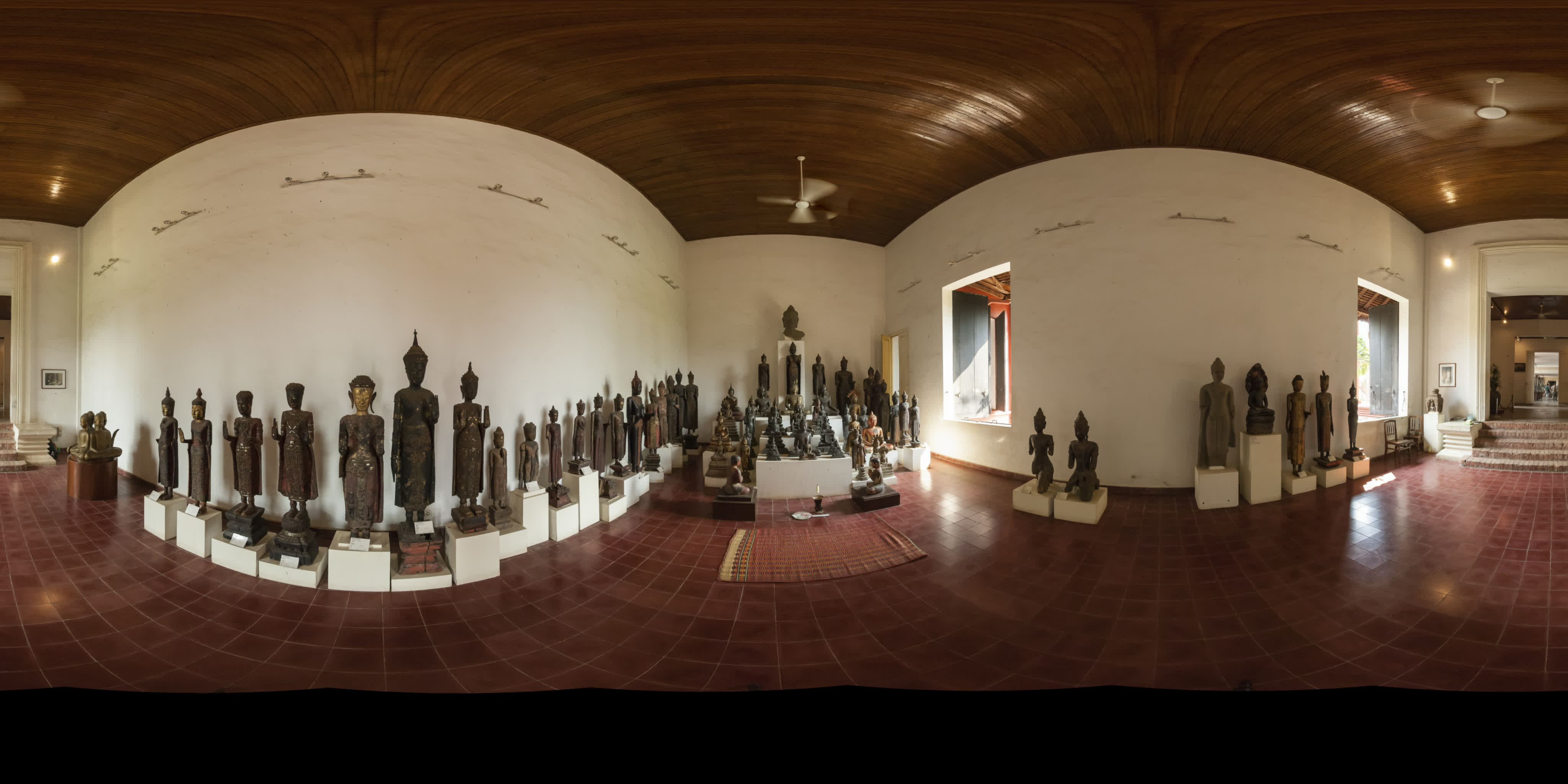## v360 ball 1 - animate yaw (with bash)

The v360 filter has many projection formats, here the "ball" format. Seems to be a fisheye format close to 300° FOV with an alpha mask option. No practical use for a panoramic photographer, but visually interesting.

Here I animate the equirectangular file by making 360 equirectangular images with 1° horizontal increase*. Starting from the left, position -180 to 180 (the yaw range in v360).

ffmpeg v360 filter

* to increase the number of frames, I have to leave bash as it only accepts integers. I would use Black Magic Design's Fusion Studio with Reactor to directly make an intermediate movie in any length.180e.jpg
Equirectangular input (as .tif), the Buddha hall, National Museum, Phnom Penh, 2007.

Introduction to Bash Shell Scripting, Flavio Copes
Bash - Getting started with Bash
shellcheck is your friend when the shell is stubborn.

To make a batch script, use bash or zsh as follows (delete the comments if in zsh):

`#!/bin/bash`
`yaw=-180`# degrees, range -180 to 180. If you want to loop it, stop at 179.
`frame=0`# start from 0.
`while [ \$frame -lt 360 ]`# (-lt = less than) loop from 0 to 359 frames, then stop.
`do`
`ffmpeg -i a/input.tif -hide_banner -vf v360=input=e:output=e:yaw="\$yaw" b/"\$frame".jpg`
`yaw=\$((yaw + 1))`# increase yaw by one (integer).
`frame=\$((frame + 1))`# increase image number.
```done ```Then, add 00 to image 1-9, add 0 to 10-99 for the next step (or use a file renamer):

`a=1`
`for i in *.jpg; do`
`new=\$(printf "%03d.jpg" \${a})`
`echo renaming \${i} \${new}`
`mv \${i} \${new}`
`let a=a+1`
`done`

Make a high quality intermediate movie from the jpeg files in .mov (proRes422 or better) or equivalent pc format:

Set "input framerate" on still images.

` ffmpeg -r 30 -i b/%03d.jpg -c:v prores_ks -profile:v 3 -vendor apl0 -bits_per_mb 8000 -r 30 -pix_fmt yuv422p10le proRes422.mov`

eq_pan.mp4
panning movie, from the proRes422.mov

And to make the ball format .mp4 video use:

` ffmpeg -i proRes422.mov -hide_banner -vf "v360=input=e:output=ball:pitch=0:interp=cubic:w=2048:h=2048" -pix_fmt yuv420p ball.mp4 `
interp=cubic Note: more complex interpolation methods require much more memory to run. Default value is 'line'.

Output "ball.mp4", 12s at 30fps, pitch=0.
pitch=90 places zenith in the center (rotates CW), pitch=-90 places nadir in the center (rotates CCW).

h9.mp4
```	pitch=
90  68  45
22   0 -22
-45 -68 -90 ```
The v360 filter has an alpha mask option: Build mask in alpha plane for all unmapped pixels by marking them fully transparent. Boolean value, by default disabled. Set to true with "alpha_mask=1". Apart from ball, the orthographic and perspective format have unmapped pixels that produces working alpha, any more?

` ffmpeg -i proRes422.mov -hide_banner -vf "v360=input=e:output=ball:pitch=30:interp=cubic:w=2048:h=2048:alpha_mask=1" -c:v prores_ks -profile:v 4444 -alpha_bits 8 -bits_per_mb 8000 -pix_fmt yuva444p10le ball30alpha.mov `

Here I'm compositing the alpha masked video over the panning movie background:

` ffmpeg -i proRes422.mov -i ball30alpha.mov -hide_banner -filter_complex "overlay=x=W=4096:y=H=2048:x=w=2048:y=h=2048:x=1024:y=0,zscale=1080:540" -pix_fmt yuv420p -c:v libx264 -color_primaries bt709 -colorspace bt709 -color_range tv -color_trc 709 -movflags +faststart -preset slow -crf 18 ball_overlay1080f.mp4 `

ball_overlay1080f.mp4
pitch=30